# User:Tohline/SSC/Structure/WhiteDwarfs

(diff) ← Older revision | Current revision (diff) | Newer revision → (diff)

# White Dwarfs

The following summaries are drawn from Appendix A of Even & Tohline (2009).

### Chandrasekhar mass

Chandrasekhar (1935) was the first to construct models of spherically symmetric stars using the barotropic equation of state appropriate for a degenerate electron gas, namely,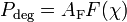$~P_\mathrm{deg} = A_\mathrm{F} F(\chi)$ where: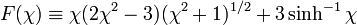$F(\chi) \equiv \chi(2\chi^2 - 3)(\chi^2 + 1)^{1/2} + 3\sinh^{-1}\chi$ and: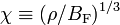$\chi \equiv (\rho/B_\mathrm{F})^{1/3}$

In so doing, he demonstrated that the maximum mass of an isolated, nonrotating white dwarf is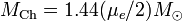$M_\mathrm{Ch} = 1.44 (\mu_e/2)M_\odot$, where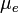$~\mu_e$ is the number of nucleons per electron and, hence, depends on the chemical composition of the white dwarf. A concise derivation of MCh (although, at the time, it was referred to as M3) is presented in Chapter XI of Chandrasekhar (1967), where we also find the expressions for the characteristic Fermi pressure,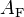$~A_\mathrm{F}$, and the characteristic Fermi density,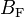$~B_\mathrm{F}$. The derived analytic expression for the limiting mass is,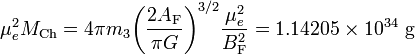$\mu_e^2 M_\mathrm{Ch} = 4\pi m_3 \biggl( \frac{2A_\mathrm{F}}{\pi G} \biggr)^{3/2} \frac{\mu_e^2}{B_\mathrm{F}^2} = 1.14205\times 10^{34} ~\mathrm{g}$,

where the coefficient,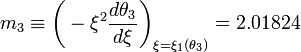$m_3 \equiv \biggl(-\xi^2 \frac{d\theta_3}{d\xi} \biggr)_\mathrm{\xi=\xi_1(\theta_3)} = 2.01824$,

represents a structural property of n = 3 polytropes (γ = 4 / 3 gasses) whose numerical value can be found in Chapter IV, Table 4 of Chandrasekhar (1967). We note as well that Chandrasekhar (1967) identified a characteristic radius,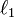$\ell_1$, for white dwarfs given by the expression,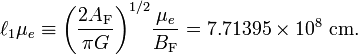$\ell_1 \mu_e \equiv \biggl( \frac{2A_\mathrm{F}}{\pi G} \biggr)^{1/2} \frac{\mu_e}{B_\mathrm{F}} = 7.71395\times 10^8~\mathrm{cm} .$

Nauenberg (1972) derived an analytic approximation for the mass-radius relationship exhibited by isolated, spherical white dwarfs that obey the zero-temperature white-dwarf equation of state. Specifically, he offered an expression of the form,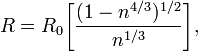$R = R_0 \biggl[ \frac{(1 - n^{4/3})^{1/2}}{n^{1/3}} \biggr] ,$

where,

 n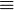$\equiv$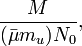$\frac{M}{(\bar{\mu} m_u) N_0} ,$ N0$\equiv$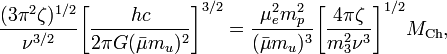$\frac{(3\pi^2\zeta)^{1/2}}{\nu^{3/2}} \biggl[ \frac{hc}{2\pi G(\bar\mu m_u)^2} \biggr]^{3/2} = \frac{\mu_e^2 m_p^2}{(\bar\mu m_u)^3} \biggl[ \frac{4\pi \zeta}{m_3^2 \nu^3} \biggr]^{1/2} M_\mathrm{Ch} ,$ R0$\equiv$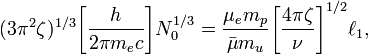$(3\pi^2 \zeta)^{1/3} \biggl[ \frac{h}{2\pi m_e c} \biggr] N_0^{1/3} = \frac{\mu_e m_p}{\bar\mu m_u} \biggl[ \frac{4\pi \zeta}{\nu} \biggr]^{1/2} \ell_1 ,$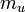$~m_u$ is the atomic mass unit,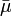$~\bar{\mu}$ is the mean molecular weight of the gas, and ζ and ν are two adjustable parameters in Nauenberg's analytic approximation, both of which are expected to be of order unity. By assuming that the average particle mass denoted by Chandrasekhar (1967) as emp) is identical to the average particle mass specified by Nauenberg (1972) as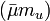$(\bar\mu m_u)$ and, following Nauenberg's lead, by setting ν = 1 and,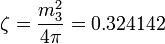$\zeta = \frac{m_3^2}{4\pi} = 0.324142$,

in the above expression for N0, we see that,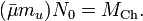$(\bar\mu m_u)N_0 = M_\mathrm{Ch} .$

Hence, the denominator in the above expression for n becomes the Chandrasekhar mass. Furthermore, the above expressions for R0 and R become, respectively,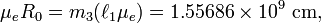$\mu_e R_0 = m_3(\ell_1 \mu_e) = 1.55686\times 10^9~\mathrm{cm} ,$

and,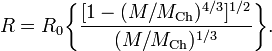$R = R_0 \biggl\{ \frac{[1 - (M/M_\mathrm{Ch})^{4/3} ]^{1/2}}{(M/M_\mathrm{Ch})^{1/3}} \biggr\} .$

Finally, by adopting appropriate values of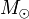$M_\odot$ and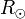$R_\odot$, we obtain essentially the identical approximate, analytic mass-radius relationship for zero-temperature white dwarfs presented in Eqs. (27) and (28) of Nauenberg (1972):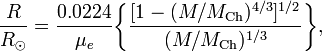$\frac{R}{R_\odot} = \frac{0.0224}{\mu_e} \biggl\{ \frac{[1 - (M/M_\mathrm{Ch})^{4/3} ]^{1/2}}{(M/M_\mathrm{Ch})^{1/3}} \biggr\} ,$

where,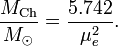$\frac{M_\mathrm{Ch}}{M_\odot} = \frac{5.742}{\mu_e^2} .$

Verbunt & Rappaport (1988) introduced the following approximate, analytic expression for the mass-radius relationship of a "completely degenerate$\ldots$ star composed of pure helium" (i.e., μe = 2), attributing the expression's origin to Eggleton (private communication):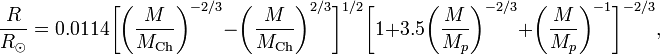$\frac{R}{R_\odot} = 0.0114 \biggl[ \biggl(\frac{M}{M_\mathrm{Ch}}\biggr)^{-2/3} - \biggl(\frac{M}{M_\mathrm{Ch}}\biggr)^{2/3} \biggr]^{1/2} \biggl[ 1 + 3.5 \biggl(\frac{M}{M_p}\biggr)^{-2/3} + \biggl(\frac{M}{M_p}\biggr)^{-1} \biggr]^{-2/3} ,$

where Mp is a constant whose numerical value is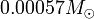$0.00057 M_\odot$. This "Eggleton" mass-radius relationship has been used widely by researchers when modeling the evolution of semi-detached binary star systems in which the donor is a zero-temperature white dwarf. Since the Nauenberg (1972) mass-radius relationship discussed above is retrieved from this last expression in the limit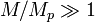$M/M_p \gg 1$, it seems clear that Eggleton's contribution was the insertion of the term in square brackets involving the ratio M / Mp which, as Marsh, Nelemans & Steeghs (2004) phrase it, "allows for the change to be a constant density configuration at low masses (Zapolsky & Salpeter 1969)."

## Highlights from Discussion by Shapiro & Teukolsky (1983)

Here we interleave our own derivations and discussions with the presentation found in [ST83].

In our accompanying discussion, we have shown that the equilibrium radius of an isolated polytrope is given, quite generally, by the expression,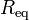$~R_\mathrm{eq}$$~=$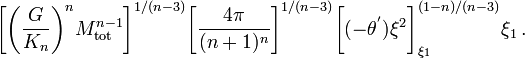$~\biggl[\biggl(\frac{G}{K_n}\biggr)^n M_\mathrm{tot}^{n-1} \biggr]^{1/(n-3)} \biggl[ \frac{4\pi}{(n+1)^n} \biggr]^{1/(n-3)} \biggl[(-\theta^') \xi^2\biggr]_{\xi_1}^{(1-n)/(n-3)} \xi_1 \, .$

Inverting this provides the following expression for the total mass in terms of the equilibrium radius: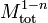$~ M_\mathrm{tot}^{1-n}$$~=$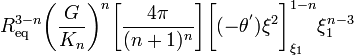$~R_\mathrm{eq}^{3-n}\biggl(\frac{G}{K_n}\biggr)^n \biggl[ \frac{4\pi}{(n+1)^n} \biggr] \biggl[(-\theta^') \xi^2\biggr]_{\xi_1}^{1-n} \xi_1^{n-3}$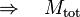$~\Rightarrow~~~ M_\mathrm{tot}$$~=$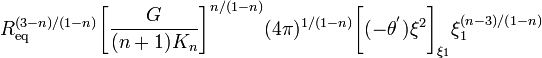$~R_\mathrm{eq}^{(3-n)/(1-n)}\biggl[\frac{G}{(n+1)K_n}\biggr]^{n/(1-n)} ( 4\pi )^{1/(1-n)} \biggl[(-\theta^') \xi^2\biggr]_{\xi_1} \xi_1^{(n-3)/(1-n)}$$~=$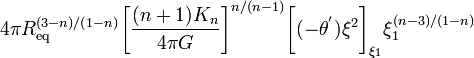$~4\pi R_\mathrm{eq}^{(3-n)/(1-n)}\biggl[\frac{(n+1)K_n}{4\pi G}\biggr]^{n/(n-1)} \biggl[(-\theta^') \xi^2\biggr]_{\xi_1} \xi_1^{(n-3)/(1-n)}$

As is shown by the following boxed-in equation table, this expression matches equation (3.3.11) from [ST83], except for the sign of the exponent on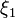$~\xi_1$, which is demonstratively correct in our expression.

Equations extracted from §3.3 (p. 63) and §2.3 (p. 27) of Shapiro & Teukolsky (1983)

"Black Holes, White Dwarfs, and Neutron Stars:   The Physics of Compact Objects"

(New York: John Wiley & Sons)$~M$$~=$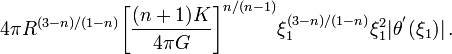$~ 4\pi R^{(3-n)/(1-n)} \biggl[ \frac{(n+1)K}{4\pi G} \biggr]^{n/(n-1)} \xi_1^{(3-n)/(1-n)} \xi_1^2 |\theta^'(\xi_1)| \, .$ (Eq. 3.3.11)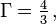$~\Gamma = \tfrac{4}{3} \, ,$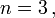$~n = 3 \, ,$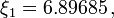$~\xi_1 = 6.89685 \, ,$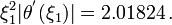$~\xi_1^2|\theta^'(\xi_1)| = 2.01824 \, .$               (Eq. 3.3.12)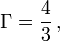$~\Gamma = \frac{4}{3} \, ,$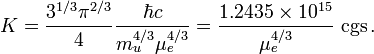$~K = \frac{3^{1 / 3} \pi^{2 /3}}{4} \frac{\hbar c}{m_u^{4 / 3}\mu_e^{4 / 3}} = \frac{1.2435 \times 10^{15}}{\mu_e^{4 /3}} ~\mathrm{cgs} \, .$                   (Eq. 2.3.23)

Each equation has been retyped here exactly as it appears in the original publication.

Given that (see equation 3.3.12 of [ST83]; see the boxed-in equation table) in the relativistic limit,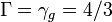$~\Gamma = \gamma_g = 4/3$ — that is,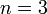$~n=3$ — and acknowledging as we have above that, for isolated$~n = 3$ polytropes,$m_3 \equiv \biggl(-\xi^2 \frac{d\theta_3}{d\xi} \biggr)_\mathrm{\xi=\xi_1(\theta_3)} = 2.01824$,

this polytropic expression for the mass becomes,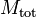$~ M_\mathrm{tot}$$~=$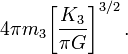$~4\pi m_3 \biggl[\frac{ K_3 }{\pi G}\biggr]^{3/2} \, .$

Separately, [ST83] show that the effective polytropic constant for a relativistic electron gas is (see their equation 2.3.23, reprinted above in the boxed-in equation table),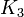$~K_3$$~=$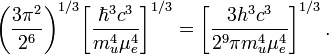$~ \biggl( \frac{3\pi^2}{2^6}\biggr)^{1/ 3} \biggl[ \frac{ \hbar^3 c^3 }{ m_u^4 \mu_e^4 } \biggr]^{ 1 / 3} = \biggl[ \frac{3 h^3 c^3 }{2^9\pi m_u^4 \mu_e^4 } \biggr]^{ 1 / 3} \, .$

Together, then, the [ST83] analysis gives,$~ M_\mathrm{tot}$$~=$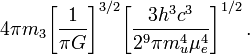$~4\pi m_3 \biggl[\frac{ 1 }{\pi G}\biggr]^{3/2} \biggl[ \frac{3 h^3 c^3 }{2^9\pi m_u^4 \mu_e^4 } \biggr]^{ 1 / 2} \, .$

Given that the definitions of the characteristic Fermi pressure,$~A_\mathrm{F}$, and the characteristic Fermi density,$~B_\mathrm{F}$, are,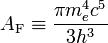$A_\mathrm{F} \equiv \frac{\pi m_e^4 c^5}{3h^3}$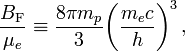$\frac{B_\mathrm{F}}{\mu_e} \equiv \frac{8\pi m_p}{3} \biggl( \frac{m_e c}{h} \biggr)^3 \, ,$

we have,$~ M_\mathrm{tot}$$~=$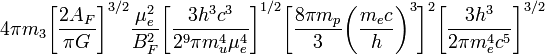$~4\pi m_3 \biggl[\frac{ 2A_F }{\pi G}\biggr]^{3/2} \frac{\mu_e^2}{B_F^2} \biggl[ \frac{3 h^3 c^3 }{2^9\pi m_u^4 \mu_e^4 } \biggr]^{ 1 / 2} \biggl[ \frac{8\pi m_p}{3} \biggl( \frac{m_e c}{h} \biggr)^3 \biggr]^2 \biggl[\frac{3h^3}{2\pi m_e^4 c^5} \biggr]^{3 / 2}$$~=$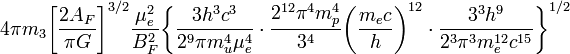$~4\pi m_3 \biggl[\frac{ 2A_F }{\pi G}\biggr]^{3/2} \frac{\mu_e^2}{B_F^2} \biggl\{ \frac{3 h^3 c^3 }{2^9\pi m_u^4 \mu_e^4 } \cdot \frac{2^{12} \pi^4 m_p^4}{3^4} \biggl( \frac{m_e c}{h} \biggr)^{12} \cdot \frac{3^3h^9}{2^3\pi^3 m_e^{12} c^{15}} \biggr\}^{1 / 2}$$~=$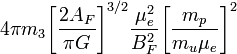$~4\pi m_3 \biggl[\frac{ 2A_F }{\pi G}\biggr]^{3/2} \frac{\mu_e^2}{B_F^2} \biggl[ \frac{ m_p }{m_u \mu_e } \biggr]^{2}$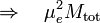$~\Rightarrow ~~~ \mu_e^2 M_\mathrm{tot}$$~=$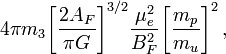$~4\pi m_3 \biggl[\frac{ 2A_F }{\pi G}\biggr]^{3/2} \frac{\mu_e^2}{B_F^2} \biggl[ \frac{ m_p }{m_u } \biggr]^{2} \, ,$

which matches the expression presented above for the Chandrasekhar mass if we set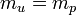$~m_u = m_p$.

 … work by Roxburgh (1965, Z. Astrophys., 62, 134), Anand (1965, Proc. Natl. Acad. Sci. U.S., 54, 23), and James (1964, ApJ, 140, 552) shows that the [Chandrasekhar (1931, ApJ, 74, 81)] mass limit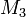$~M_3$ is increased by only a few percent when uniform rotation is included in the models, … In this Letter we demonstrate that white-dwarf models with masses considerably greater than$~M_3$ are possible if differential rotation is allowed … models are based on the physical assumption of an axially symmetric, completely degenerate, self-gravitating fluid, in which the effects of viscosity, magnetic fields, meridional circulation, and relativistic terms in the hydrodynamical equations have been neglected.